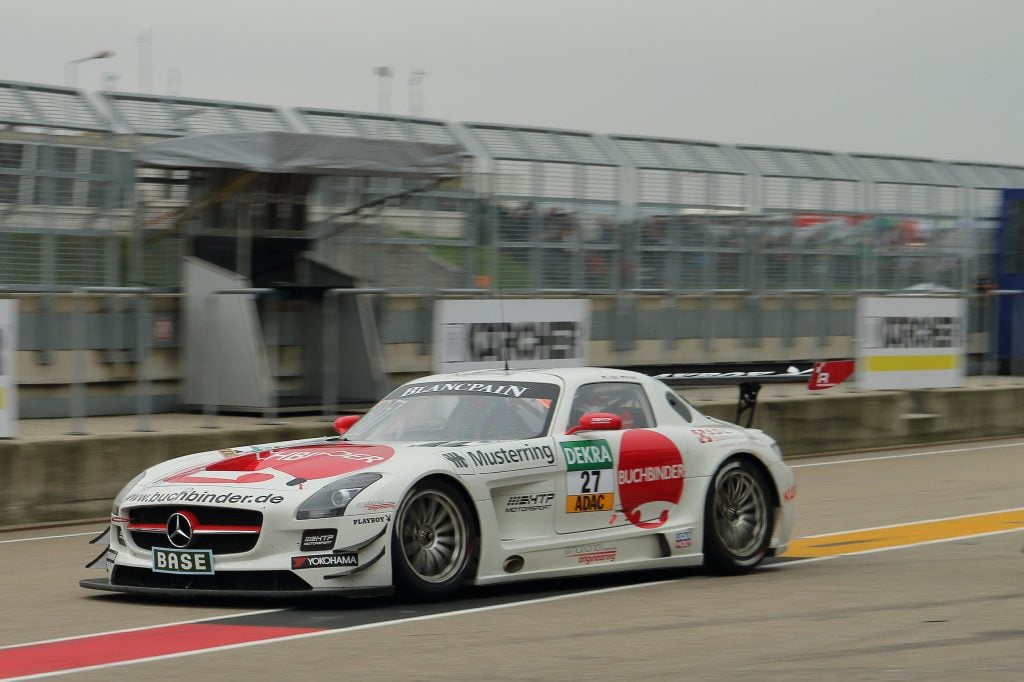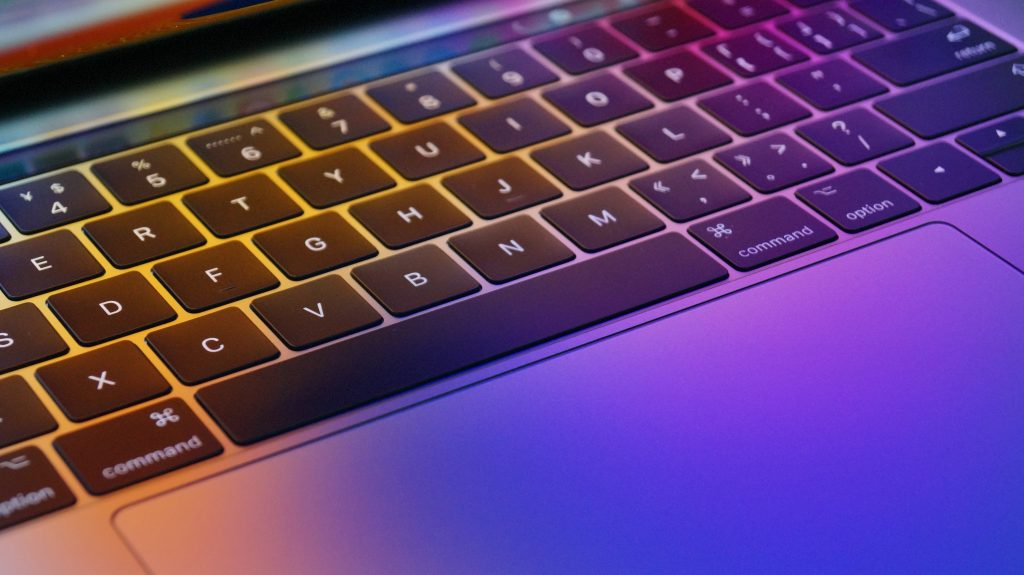# How to Use a Tachymeter Bezel on a Chronograph Watch?

If you have a watch with a chronograph, it may have a tachymeter scale on the bezel. The tachymeter scale adds some very handy functionality to your watch, allowing you to calculate several different things:

• How fast something is going, based on how long it takes to cover a certain distance.
• How far something has travelled, based on how fast it is travelling over a certain time.
• How many units of any task can be performed over the course of an hour.

In order to perform any of these calculations with the help of the tachymeter, it is vital that the speed involved be constant.

This is true whether we are referring to the speed of a physical object travelling over a certain distance in space, or the speed at which a task is being performed.

The tachymeter scale is based on this formula:

T = 3600 / t

T represents the value on the tachymeter scale.

t refers to how many seconds have elapsed as an event unfolds.

3600 is simply how many seconds there are in an hour.

Interestingly, it does not matter whether you want an answer in “miles per hour,” “kilometres per hour,” “leagues per hour,” or any other rate unit. You can get the answer you are looking for regardless.

To understand how this works, it is best to look at a few examples. This will help you to see how you can make use of the tachymeter on your watch.

## Example #1: Measuring SpeedImagine that you are at a racetrack, watching a car make its rounds at a constant speed.

You do not know what that speed is, but you do know that it is not varying. You also know the distance around the track happens to be a mile.

This is a situation where you can calculate speed with the aid of your tachymeter. Here is how to do it.

1. When the driver starts the loop, start your chronograph.
2. When the driver completes the loop, stop your chronograph.
3. Check the dial to see where the second hand has stopped. For this example, let us imagine that it has stopped at 40 seconds.
4. Now, simply check what number this aligns with on the tachymeter. The number you see is 90.

That’s it! You have calculated that the driver completed the one-mile loop at 90 miles per hour.

You could do the same thing with a one-kilometre loop, and get the answer in kilometres per hour. Again, any units are fine.

## Example #2: Measuring DistanceJust as you can measure speed using a tachymeter, you can measure distance given that you have the proper setup.

Once more, the rate of travel must be constant. In this case, you will need to know the speed.

Let us imagine that you are watching a driver that you know is going at 90 miles per hour.

You want to know when he has driven one mile.

1. Start your chronograph at the point you wish to measure the distance from (for example, the start of a racetrack loop). Call this Point A.
3. At this point, take note of the driver’s position. Call this Point B.

The distance between Point A and Point B is one mile.

There is an important limitation to take note of. If an event takes more than 60 seconds to unfold (for example, a car is going 50 miles per hour rather than 60 miles per hour or more), the tachymeter cannot be used without altering the math.

Thankfully, the modification to the process is simple:

• Before you start your chronograph, multiply the speed by two. This makes it possible to use the tachymeter as usual.
• After you stop your chronograph and observe the distance that the driver has covered, divide that distance by two.

So what you will be looking at is a half mile, rather than a mile. But you have still successfully measured the distance the driver covered over a certain span of time at 90 miles per hour.

## Example #3: Measuring EfficiencyA third thing you can do is measure the efficiency of a task. It can literally be any task you want.

As with the examples above, we must assume that the rate at which the task is performed does not vary.

Let’s say that your teacher has assigned you a 5,000-word term paper.

You have already conducted your research, put an outline together, and now need merely to type up the paper.

You feel confident that your speed of typing will not range much. You want to calculate how many hours it will take you to complete the paper.

1. Start the chronograph and begin typing. Obviously, you need more than one word to get a gauge on time, so type some amount of words that will be easy to do math with, like 25.
2. Stop the chronograph after typing the 25 words.
3. Note the time on the chronograph and the number on the tachymeter. Let’s say 30 seconds have passed, and the tachymeter reads 120.

This implies that you can type 120 twenty-five-word units over the course of an hour.

120 x 25 = 3,000 words. You will manage 3,000 words of your paper within an hour. You should need less than two hours to complete the entire paper.

## The Tachymeter Concept Can Be Tricky to Grasp at First, But the Steps Are Easy

If you do not have a head for numbers, you may find the tachymeter challenging to understand initially. But the process to use it to calculate time, distance or efficiency is quite simple and straightforward. Indeed, the formula which allows you to use the tachymeter to do calculations with any unit you require is quite elegant. This makes the tachymeter bezel a tool that like the watch itself is both useful and beautiful.##### Vintage Watch Inc

Dennis is the founder and editor of Vintage Watch Inc. Passionate about Soviet and Japanese vintage timepieces and a finance professional by day, he proudly wears a Seiko Pogue with his suit.